# Sudoku techniques & skills & rules | Suduku solver

Sudoku is a classic logic game and a number puzzle. In classic Sudoku, the objective is to fill a 9 × 9 grid with numbers 1-9 so that each column, each row, and each of the nine 3 × 3 subgrids that compose the grid contain all of the digits from 1 to 9. The puzzle setter provides a partially completed grid, which for a well-posed puzzle has a single solution.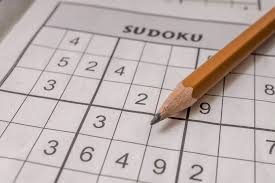### How to play Sudoku?

The enormous popularity of sudoku across the world is due to a couple of simple facts:

• The rules of Sudoku are easy to learn.
• Sudoku puzzles vary in difficulty from simple to devious.
• Puzzles have a unique solution that can be arrived at using pure logic. No guessing is required.
• Sudoku is not a math game but rather about identifying logical patterns.
• Complex strategies must be utilized in order to solve the hardest puzzles.

### Sudoku techniques & skills

1. Scanning Skills(Row scanning/Column scanning/Block scanning)

The easiest trick to get started with Sudoku puzzles is to scan all rows, all columns, and all grids, eliminating numbers or squares and finding the only number that fits in a certain square. For solving simple Sudoku problems, the scanning technique is the fastest and most effective shortcut. However, the scanning technique is not very effective for some difficult Sudoku puzzles. Here are some examples that introduce the scanning technique.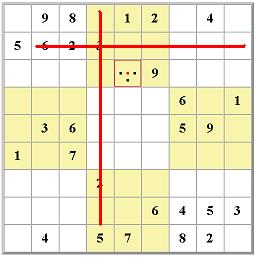2. Unique Candidate

You know that each block, row and column on a Sudoku board must contain every number between 1 and 9. Therefore, if a number, say 4, can only be put in a single cell within a block/column/row, then that number is guaranteed to fit there. This example illustrates the number 4 as the unique candidate for the cell marked in red.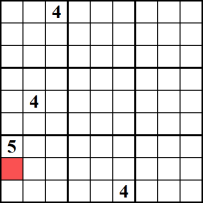3. Sole Candidate

When a specific cell can only contain a single number, that number is a “sole candidate”. This happens whenever all other numbers but the candidate number exists in either the current block, column, or row. In this example, the red cell can only contain the number 5, as the other eight numbers have all been used in the related block, column and row.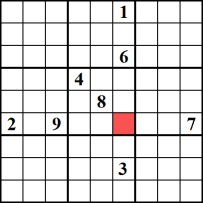4. Naked Subset

The example shows that row number 1 and row number 5 both have a cell in the same column containing only candidate numbers 4 and 7. These two numbers appear as candidates in all of the other open cells in that column too, but since they are the only two candidates in rows 1 and 5, these two numbers cannot appear anywhere else in the row, thus you can remove them. In the example, the two candidate pairs circled in red, are the sole candidates. Since 4 and 7 must be placed in either of these two cells, all of the pairs circled in blue can remove those numbers as candidates. In this puzzle, this means 1 becomes the sole candidate in the second row; 2 becomes the sole candidate in row 6; and thus, 6 is the sole candidate for row number 4.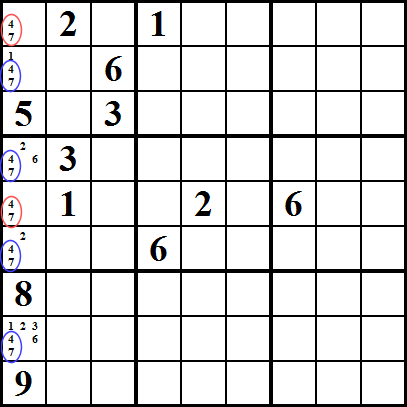5. Hidden subset

This is similar to the Naked subset, but it affects the cells holding the candidates. In this example, we see that the numbers 5, 6, 7 can only be placed in cells 5 or 6 in the first column (marked in a red circle) and that the number 5 can only be inserted in cell number 8 (marked in a blue circle). Since 6 and 7 must be placed in one of the cells with a red circle, it follows that the number 5 has to be placed in cell number 8, and thus we can remove any other candidates from the 8th cell; in this case, 2 and 3.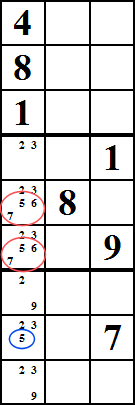6. X-Wing

This method can work when you look at cells comprising a rectangle, such as the cells marked in red. In this example, let’s say that the red and blue cells all have the number 5 as candidate numbers. Now, imagine if the red cells are the only cells in columns 2 and 8 in which you can put 5. In this case, you obviously need to put a 5 in two of the red cells, and you also know they cannot both be in the same row. Well, now, this means you can eliminate 5 as the candidate for all the blue cells. This is because, in the top row, either the first or the second red cell must have a 5, and the same can be said about the lower row.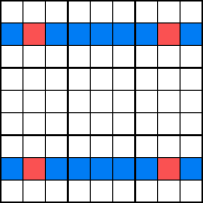7. Swordfish

Swordfish is a more complicated version of X-Wing. In most cases, the technique might seem like much work for very little pay, but some puzzles can only be solved with it. So if you want to be a sudoku-solving master, read on!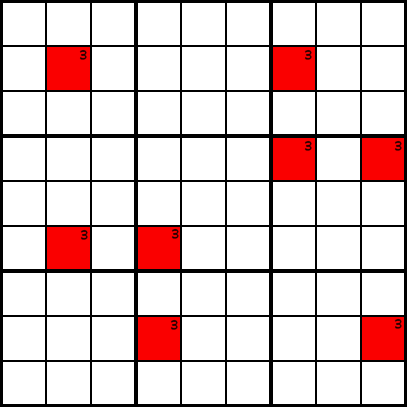Example A

In example A, we’ve plotted in some candidate cells for the number 3. Now, assume that in columns 2, 4, 7, and 9, the only cells that can contain the number 3 are the ones marked in red. You know that each column must contain a 3.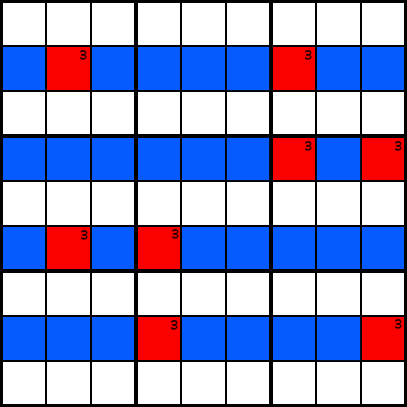Example B

Look at example B. We can eliminate 3 candidates in every cell marked in blue. The reason for this is that if we consider the possible placements of the number 3 in the red cells, we get two alternatives: either you must put 3s in the green cells, or in the purple cells, as example C shows. In any case, each of columns 2, 4, 7, and 9, must contain a 3 in one of the colored cells, so no other cell in those rows can contain a 3.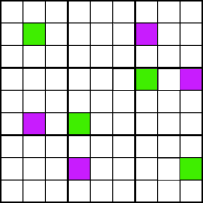Example C

How do you recognize a swordfish pattern? You look for cells with common candidate numbers that can be chained together like in example D. If you start on, say, the top-left red cell. Then you draw a line either vertically or horizontally until you reach another cell containing the same candidate number. Then you repeat this pattern until you return to the original cell. If you reach the original cell, you have a swordfish pattern!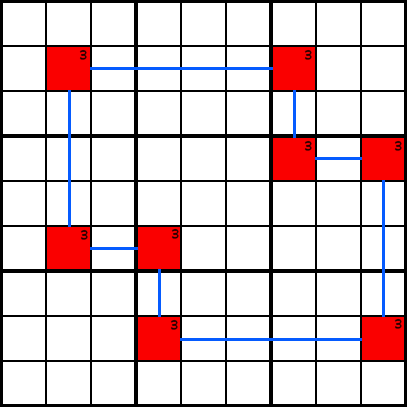Example D

Here are the most used Sudoku techniques & skills, hope it is helpful to you. If you want to play Sudoku online, you can also download Sudoku – Number match game. Sudoku – Number match game is a free & popular classic number puzzle game to train your brain and logic. Solve daily Sudoku to learn and improve your skills with Sudoku – Number match game and have fun!